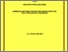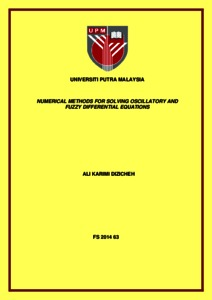# Numerical methods for solving oscillatory and fuzzy differential equations

## Citation

Dizicheh, Ali Karimi (2014) Numerical methods for solving oscillatory and fuzzy differential equations. Doctoral thesis, Universiti Putra Malaysia.

## Abstract

In this thesis we develop ¯ve numerical schemes for solving ordinary di®erential equations. These include exponentially-¯tted Runge-Kutta method, trigonometri- cally ¯tted hybrid method, Legendre wavelet method on large intervals as well as an iterative spectral collocation method, exponentially-¯tted fuzzy Runge-Kutta method, exponentially-¯tted system of fuzzy Runge-Kutta method. The stability analysis, estimation of local truncation errors and the e±ciency of the methods' implementation in computer programs are discussed. An exponentially-¯tted explicit Runge-Kutta method of algebraic order 4 is for- mulated for the ¯rst-order ordinary di®erential equations y0 = f(x; y); y(x0) = y0: It integrates exactly the ¯rst-order systems where their solutions are expressed as linear combinations of fexp (wx); exp (¡wx)g or fcos (¸x); sin(¸x)g where w = ¸i. Stability analysis of our approach as well as a good estimation for the local trunca- tion errors are presented. The e±ciency of the exponentially-¯tted Runge-Kutta method is tested via some numerical experiments and a comparison with other existing methods. A trigonometrically ¯tted explicit hybrid three-stage method is derived for the second-order initial value problems with oscillatory solutions. We compare our results with the classical hybrid method and the trigonometrically ¯tted explicit Runge-Kutta method through several examples. Our results indicate that trigono- metrically ¯tted explicit hybrid method is more e±cient than the classical hybrid method. we analyze the stability, phase- lag (dispersion) and dissipation. An iterative spectral collocation method are introduced for solving initial value problems de¯ned on large intervals. Indeed, the Legendre wavelet method is ex- tended and proved valid for large interval. Then, the Legendre-Guass collocation points of the Legendre wavelets are computed. By employing an interpolation based on Legendre wavelet, we ¯nd approximate solution for any order (¯rst-order and second-order) di®erential equations. Using this strategy the iterative spectral method converts the di®erential equation to a set of algebraic equations. Solving this set of algebraic equations yields an approximate solution. Using exponentially-¯tted Runge-Kutta (EFRK) method, we develop a method for numerically solving fuzzy ¯rst order linear and nonlinear di®erential equations under generalized di®erentiability. In addition, this method is applied for the sys- tem of ¯rst order fuzzy di®erential equations with uncertainty. The generalized Hukuhara di®erentiability are applied to estimate the solutions. For solving the fuzzy problems, the exponentially-¯tted Runge-Kutta method is applied. Finally, some examples are solved to illustrate our proposed approaches. The results are compared with those in the literature. We show that our proposed methods are simple and more accurate than the other existing methods.Preview
Text
FS 2014 63 - IR.pdfView Item